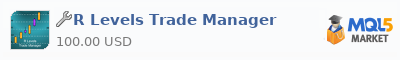### IntroductionThe Trade Manager is action with the break-even triggered at 2R and the trailing stop triggered at 4R

### Input Parameters

BE_R: the R level at which the stop-loss is moved to break-even. Note if you don’t want to use this functionality then simply make this a very large value

TrlStart_R: the R level at which the trailing stop activates. Note if you don’t want to use this functionality then simply make this a very large value

TrlSize_R: this is the amount in R’s by which the stop-loss trails behind the market. So a value of 1.5 will trail the stop-loss 1.5 times the initial risk behind the extreme of the move so far

TrlAdj_R: this is the “granularity” of the adjustments to the trailing stop. So with a value of 0.5R here then the stop-loss will only be adjusted every 0.5R and in between this amount no adjustment will be made. If this is set to 0.0 then the stop will be adjusted immediately for every new extreme in the market move.

StopType: this is either “Intrabar” or “OnClose”. If the former is selected then every tick the stop is checked to see if it needs adjusting. If the latter is selected then adjustments are only made on the close of each bar

ShowEntryAndStop: if set to true then the EA will draw in the entry and initial stop-loss levels as part of the drawing of the R levels.

ShowRLevels: if set to true then the R levels for the trade will be plotted.

RInterval: this specifies the increment in the R levels for when they are drawn

RLast: this specified the last R value for when they are drawn

NumBarsWidth: this specifies the length of the R-levels plots (in bars) that the lines are drawn

DeleteLinesOnTradeClose: if set to true then the plot lines are removed once the trade is closed. If set to false then they will remain on the chart after the end of the trade

DeleteLinesOnEARemoval: if set to true then the plot lines are removed once the EA is removed from the chart. If set to false then they will remain on the chart after the EA is removed.

LineThickness: this specifies the thickness of the R level plot lines

Line_Colour: this specifies the colour of the R level plot lines

LineStyle: this specifes the style (e.g. solid, dotted etc.) of the R level plot lines

Font_size: this specifies the size of the font that is used to label the R level plot lines

Font_type: this specifies the font that is used to label the R level plot lines

Font_Colour: this specifies the colour of the font that is used to label the R level plot lines

LevelLabelOffset: this specifies where the label is drawn in relation to the R level plot lines